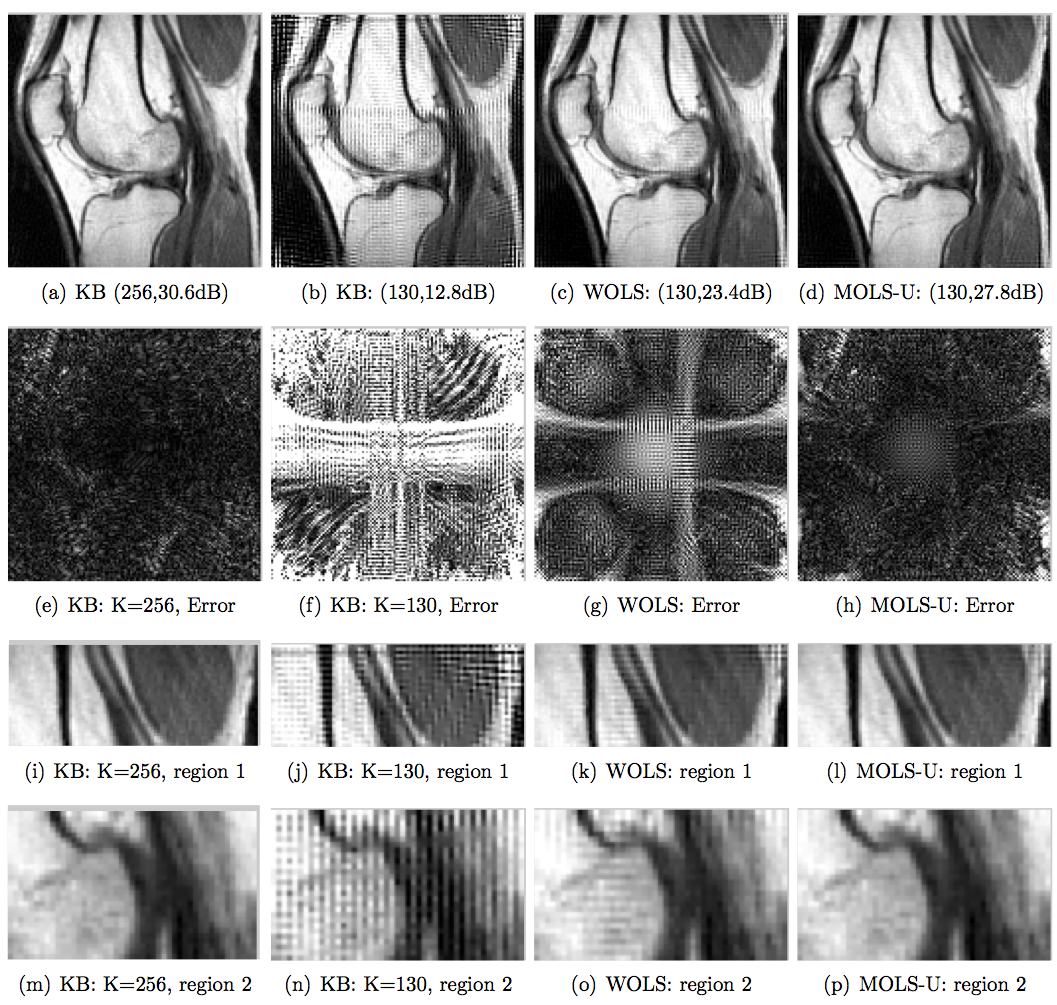# Accurate NUFFT using optimized interpolator and scale factors

Installation: Uncompress the above folder and run the demo functions Demo_NUFFT_1_knee.m and Demo_NUFFT_2_brain.m. These programs will replicate the results in the references below.

Motivation:  The main motivation is to optimize the NUFFT interpolator and the scale factors jointly to improve the accuracy of the NUFFT approximation. Classical schemes are designed to provide reasonable approximations in the two-fold oversampled (K=2N) regime. However, the resulting memory demand (4/8 fold for 2-D/3-D) implementations is often un-acceptable for implentation on GPU devices with limited onboard memory. The proposed optimal approximations considerably reduces the approximation error, thus providing similar image quality for the K~N setting.

IllustrationDemonstration of the optimal NUFFT schemes (MOLS-U and WOLS) in the context of recovering a knee image from its radial samples. The different rows correspond to the reconstructions, the errors, and zoomed reconstructions from regions in the top and middle portions of the image, respectively. The first two columns correspond to reconstruction with the classical K=2N (4 times more memory demand than the original image), the reconstruction using the classical Kaiser Bessel kernel with K=N+2 (~ the same memory demand as the original image). The last two columns correspond to K=N+2 reconstructions using the proposed worst-case ( WOLS) and mean square optimal (MOLS-U) NUFFT schemes. Note that the MOLS-U scheme provides reconstructions that are quite comparable to the classical K=2N scheme.